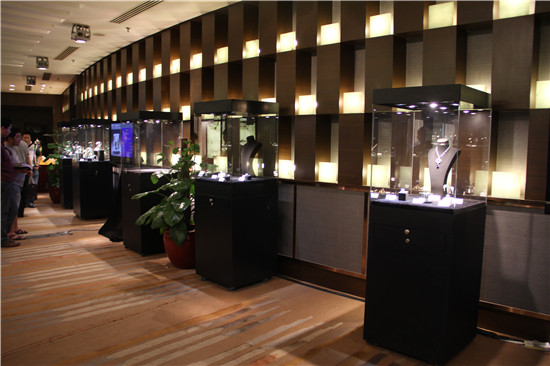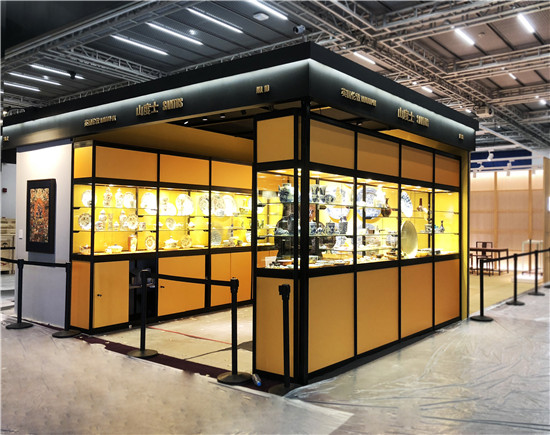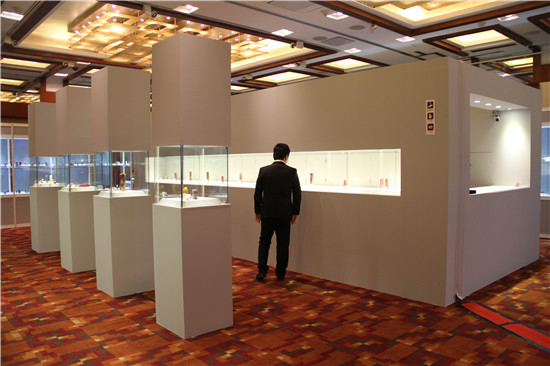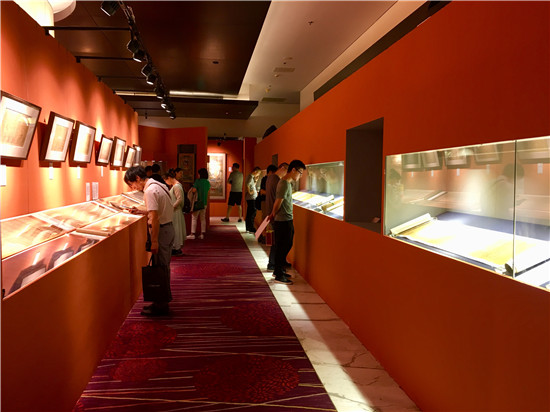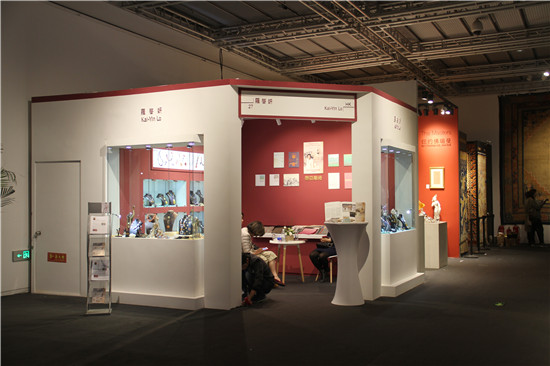400-057-5577

# 定制展柜--艺术展拍卖会博物馆专用展柜租赁销售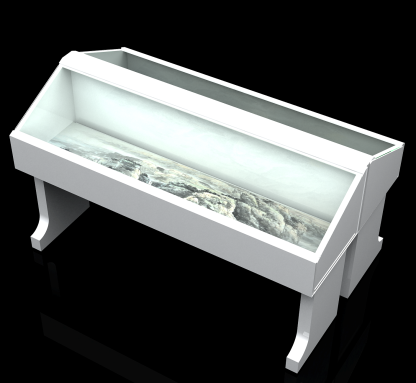（尺寸、颜色可定制）
4米LED灯带

2m(L)x0.6m(w)x1.1m(H)

1.95m(L)x0.55m(w)x0.18m(H)底部无储物空间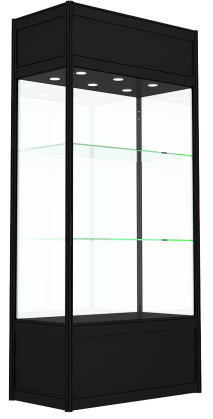LED面光灯6盏

1m(L)x0.5m(w)x2.5m(H)

0.97m(L)x0.47m(w)x1.4m(H)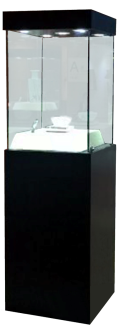（尺寸、颜色可定制）
LED面光灯5盏

0.60m(L)x0.60m(w)x2.2m(H)

0.6m(L)x0.6m(w)x0.9m(H)  底部可改造储物空间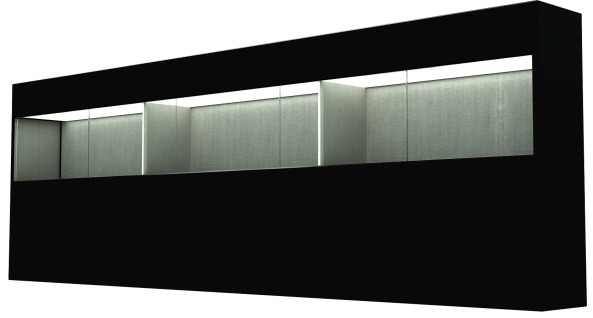（尺寸、颜色可定制）
LED面光灯10盏

1m(L)x0.6m(w)x2m(H)

0.8m(L)x0.5m(w)x0.8m(H)   底部可改造储物空间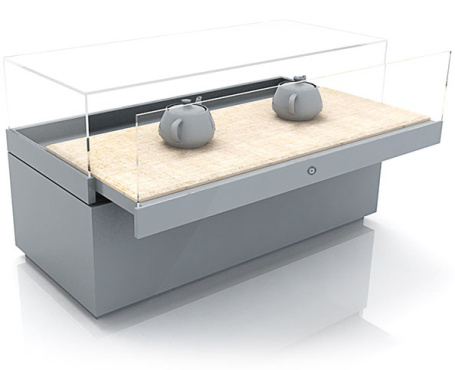（尺寸、颜色可定制）
3盏立式LED射灯

1.0 m(L)x0.6m(W)x1.2m(H)

0.8 m(L)x0.4m(W)x0.5m(H) 底部可改造储物空间